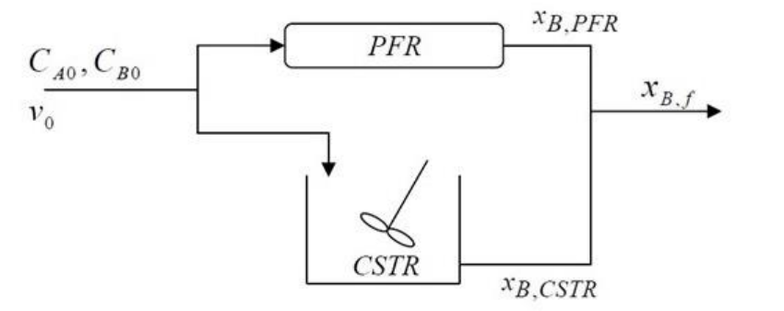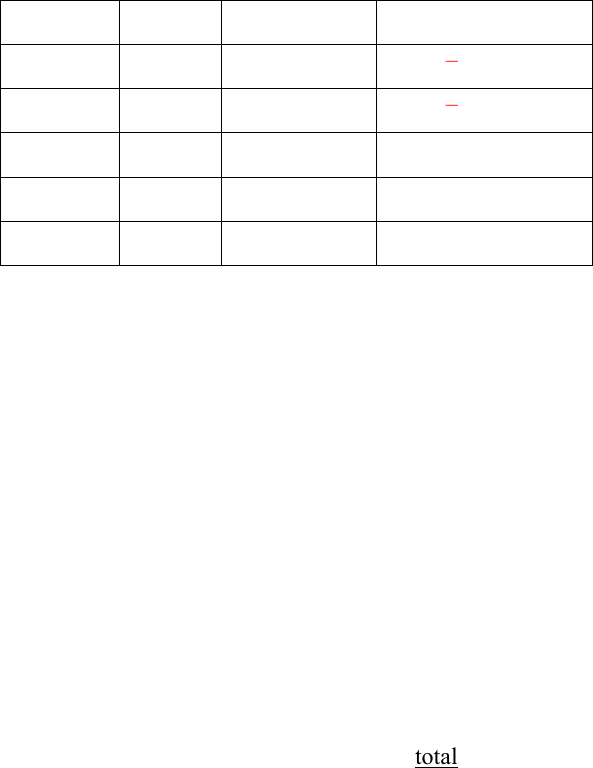# CHM ENG 142 Study Guide - Midterm Guide: Volumetric Flow Rate, Reaction Step, Limiting Reagent

27 views6 pages
School
Berkeley
Department
Chemical and Biomolecular Engineering
Course
CHM ENG 142
ProfessorChemical Engineering 142
First Midterm Exam (Spring 2020)
February 26, 2020
Problem 1 (35 points)
An inlet gas stream (v0 = 10 liter/min inlet volumetric flow rate and total concentration CT0 = 10
mol/liter) composed of A and B each at a mole fraction of 0.2 with the rest consisting of an inert
I enters the system shown below. A splitter sends 6 liter/min of the inlet stream to the PFR and 4
liter/min to the CSTR. Both reactors are isothermal and isobaric.
1.5 A + B
C + D
r = k CB k = 3 min-1
a. Is this reaction an elementary step? State at least two reasons for your conclusion.
No, this is not an elementary step.
1. Fractional molecules cannot react in a single irreducible step.
2. The reaction stoichiometry is not reflected in the rate law.
b. What is the limiting reactant? What is the inlet molar rate of B entering the CSTR?
The limiting reactant is A. Although the inlet contains equal amounts of A and B, more
moles of A are needed for each reaction event, so A will be depleted first.
FB0 = yB0(FT0) = yB0(v0CT0) = 0.2 (4 L/min * 10 mol/L) = 8 mol/min B entering the CSTR
Unlock document

This preview shows pages 1-2 of the document.
Unlock all 6 pages and 3 million more documents.c. Starting from a general mole balance, derive the steady-state design equation for a CSTR.
Calculate the volume required (VCSTR) to convert 40% of the molar rate of B entering the
CSTR (XB,CSTR = 0.4)
General mole balance: FB0 FB + rB dV = dNB/dt
For a CSTR: FB0 FB + rBV = 0 assuming steady state (no accumulation) and perfect
mixing (concentrations, and therefore rate, are the same throughout the reactor).
Species
Initial
Change
Final
A
FA0
- 1.5 XBFB0
FB0(1 1.5 XB)
B
FB0
- XBFB0
FB0(1 XB)
C
0
+ XBFB0
XBFB0
D
0
+ XBFB0
XBFB0
I
FI0
0
FI0
FT = FB0 (2 + FI0/FB0 - 0.5 XB)
FT0 = FB0 (2 + FI0/FB0)
Therefore v/v0 = FT/FT0 = 1 - XB [0.5 / (2 + 0.6/0.2)] = 1 - 0.1 XB
Plug into equation: FB0 (XB) = k FB0/v0 (1 XB) / (1 0.1 XB) V
Move all constants to one side: kV/v0 = XB (1 0.1 XB) / (1 XB)
Plug in values: 3 min-1 / 4 L/min * V = 0.4 (1 0.04) / 0.6
Therefore V = 0.85 L.
d. What is the Damkohler number for the CSTR in part (c)?
Da = kV/v0 = 3 * 0.85 / 4 = 0.64.
e. If the fraction of the molar rate of B entering the PFR that is converted in that reactor
(XB,PFR) is 0.48, what fraction (XB,f) of the total inlet molar rate of B (combined for both
reactors) has been converted at the end of the process (in the combined exit stream)?
Initial amount of B = 0.2 * 10 L/min * 10 mol/L = 20 mol/min B
Of the 8 mol/min B that enter the CSTR, 40% are reacted, so 4.8 mol/min remain.
Of the 12 mol/min B that enter the PFR, 48% are reacted, so 6.2 mol/min remain.
The total remaining amount of B after recombination is 11 mol/min.
Therefore, XB,f = (20 11)/20 = 0.45.
Unlock document

This preview shows pages 1-2 of the document.
Unlock all 6 pages and 3 million more documents.

## Document Summary

An inlet gas stream (v0 = 10 liter/min inlet volumetric flow rate and total concentration ct0 = 10 mol/liter) composed of a and b each at a mole fraction of 0. 2 with the rest consisting of an inert. A splitter sends 6 liter/min of the inlet stream to the pfr and 4 liter/min to the cstr. 1. 5 a + b c + d r = k cb k = 3 min-1. State at least two reasons for your conclusion. No, this is not an elementary step: fractional molecules cannot react in a single irreducible step, the reaction stoichiometry is not reflected in the rate law. Although the inlet contains equal amounts of a and b, more moles of a are needed for each reaction event, so a will be depleted first. Fb0 = yb0(ft0) = yb0(v0ct0) = 0. 2 (4 l/min * 10 mol/l) = 8 mol/min b entering the cstr.